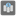• # Difference between s and data[s]

## Question related to missionBest Stock

So here is the code:

def best_stock(data):

maxprice = 0 answer = "" for s in data: print(data[s]) if data[s] > maxprice: max_price = data[s] answer = s return answer

so… if you print(data[s])

you get the integer assigned to the string

but if you print(s)

you get the string

so why is this? s is the bit of the array that is currently being looked at by the program, right? So why is it printing the string if you just ask for s and the integer if you ask for data[s]?8